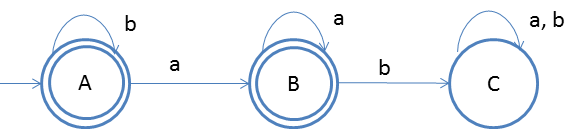# DFA String Examples

Design a DFA in which every 'a' should never followed by 'b'
Given: Input alphabet, Σ={a, b}
Language L = {ε, a, aa, aaa, ba, ...}

Clearly the language is infinite because there is infinite number of strings.The idea behind this approach is very simple, follow the steps below and you will understand.

1. Firstly for ε we will make the start sate as final state.
2. On start state if b comes then we will make self-loop as we are not violating the given property for DFA
3. If the start input comes 'a' then that state should also final state. That is why state B is a final state
4. On state B if b comes then that should be rejected, that is why state C
5. On state B if a comes then that should be accepted
6. On state C we do not care about either 'a' or 'b' that is why a self-loop
7. Here C state is a dead state

### Testing

1. Lets take one input string baa
2. Scan string from left to right
3. First input is b, so from state A we will go to state A itself
4. Second input is a, so from state A we will go to state B
5. Third input is a, so from state B we will go to state B itself(final state)
After end of the string we are at final state so string is accepted.
You may also try some examples of strings to run over this DFA, and you will understand more